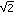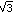# What Is a Number System? Homework

Think back to the finite system of units digits that you explored in Part A and answer the following questions about that system.

Problem H1
a. The commutative law for multiplication states that (a • b) = (b • a). Does this law hold for the finite number system in your table? Why or why not?
b. The associative law for multiplication states that (a • b) • c = a • (b • c). Does this law hold for the finite number system in your table? Why or why not?

Problem H2
a.
How could you use the multiplication table you created to divide?Think of division as a way of undoing multiplication. For example, to find y divided by x, think, “x times what number equals y?”

b. Does this finite system allow you to divide any two numbers in the system, or are there limits?

Problem H3
Is this finite set closed under the operation of multiplication?

Problem H4
The distributive law of multiplication over addition says that a • (b + c) = (a • b) + (a • c). Does the distributive law work for the finite system? Why or why not?

Problem H5
Is it possible to categorize numbers as even or odd in the finite system? Why or why not?

Problem H6
In the finite system, which numbers are multiples of 3? Which are multiples of 4? Of 5? How do you know?

Problem H7
Which numbers are perfect squares (i.e., a product of a number multiplied by itself) in the finite system? How do you know

Problem H8
In the real number system, if you multiply two numbers and you get 0, what can you conclude about these two numbers? Does the same apply in the finite number system?Think about a • b = 0, with, for example, a = 5.

Problem H9
You have determined the length ofon the number line. Can you determine the length ofon the number line?

Read these excerpts from the following book:
Seife, Charles (2000). Zero: The Biography of a Dangerous Idea (pp. 6, 12-21).

Zero: The Biography of a Dangerous Idea

### Solutions

Problem H1
a. Yes, this is true for this system, since it is true for real numbers. We can also check this from our table, which is symmetrical across its main diagonal.
b. Yes, this is true for this system, since it is true for real numbers.

Problem H2
a. If we wanted to find the answer to 83, we’d want to find a number such that when multiplied by 3 results in 8. So we are looking for solutions to the equation 3 • b = 8. To find any such number, we look across the row for 3 to find an 8. According to the table, the only place where this happens is in the intersection with the column for 6 (we’ll find the same in the column for 3 and row for 6). This means that 3 • 6 = 8, so 6 is the solution to 83.
b. There are limits. Some division problems will not work because not every number shows up in every row. For example, if we try to find 75, we are looking for a 7 in the row for 5. There is no 7 in that row, so there is no answer for this division problem.

Also, there are some division problems that give more than one answer! For example, if we try to find 86, we are looking for an 8 in the row for 6. This happens twice (in the columns for 3 and 8), so both 3 and 8 are solutions.
In either case, division is not defined for divisors 0, 2, 4, 5, 6, and 8, because you cannot get a unique answer when dividing by these numbers.

Solution H3
Yes, this system is closed under multiplication. When we multiply the units digits of two numbers, the result has a unique units digit.

Problem H4
Yes, the distributive law holds here, since it holds for real numbers.

Problem H5
Yes, it is possible to categorize numbers as even or odd. All even numbers are multiples of 2 and appear in the row and column for 2 in the multiplication table. The even numbers are 0, 2, 4, 6, and 8.

Problem H6
To find these multiples, look across the rows for 3, 4, or 5 in the multiplication table. According to the table, every number is a multiple of 3, which may seem surprising; what it means, though, is that any units digit can be the result when we multiply by 3. The set of multiples of 4 is {0, 4, 8, 2, 6}; when we multiply any whole number by 4, the units digit must be in this set. The set of multiples of 5 is {0, 5}.

Problem H7
The perfect squares are the numbers on the main diagonal of the multiplication table (0 • 0, 1 • 1, 2 • 2, etc.). According to the table, the set of perfect squares is {0, 1, 4, 9, 6, 5}. These are the only numbers that can result when we look at the units digit of any perfect square.

Problem H8
In the real number system, if the product of two numbers is 0, and we know that one of the numbers is not 0, we can be certain that the other number is 0. This, however, is not true in the finite number system. Here, 0 times any number in the system yields 0, but so do other products, such as 5 • 2, 5 • 4, or 8 • 5. (Notice that one number in such products is always 5. This is because any number multiplied by 5 will have a units digit of 0 or 5.)

Problem H9
You can find the length ofby using the Pythagorean theorem. Construct a right triangle with legs of lengths 1 and. The hypotenuse will be. Another way is to construct an equilateral triangle with side length of 2; the length of any altitude of this triangle is. The value ofis approximately 1.732.For more information on using the Pythagorean theorem, see Learning Math: Geometry, Session 6, Part C.